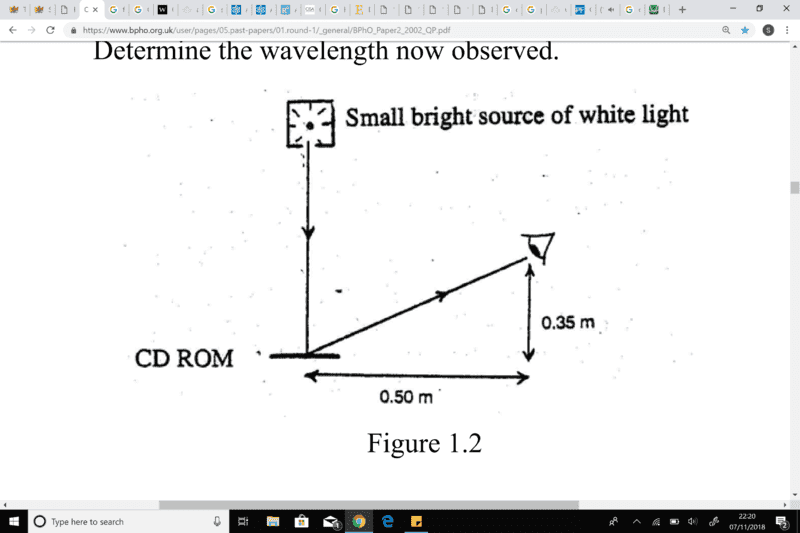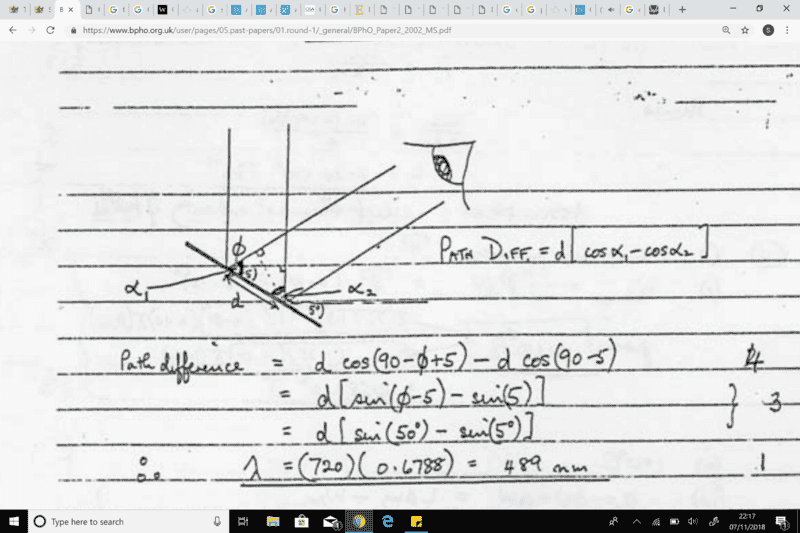# Diffraction Gratings

Sarah0001
1. A white light shines vertically down on a horizontal CD ROM disc.
The disc is viewed by a teacher whose eye is a horizontal distance 0.50 m from the disc and 0.35 m vertically above its horizontal plane, Figure 1.2. A yellow light of wavelength 590 nm is observed due to first order diffraction.. (i) Deduce the spacing d between adjacent tracks of the CD ROM.
angle subtended between incident and reflected ray is 55 degrees

2) relevant equations :This below part is what I require help with.
(ii) The CD ROM is tilted, clockwise through an angle of 5 degrees . Determine the wavelength now observed.

3. My attempt at the solution :

wavelength = 720*10^-9 * sin 60I understand that the angle of incidence ray with mirror is now 95 degrees as opposed to 90,
so 95-55 = 40
so cos theta 1 = wavelength / d , theta 1 is 40, and thus sin theta 2 = wavelength / d , theta two must be 50 degrees by ( 180-90-40).
However I do not understand why 'sin5' is subtracted by sin 50 in the worked solutions, nor do I understand how cos ( 90 - 5) leads to sin (5), or cos(90 -x+5) = sin ( x -5) for that sake
Also fundamentally I cannot understand why the worked solutions initially finds the path difference to equal to (cosine A - Cosine B ) * slit separation (d)

#### Attachments

Homework Helper
Gold Member
Normally, the incident and reflected angles are measured off of a diffraction grating as measured from normal incidence. Then the equation for constructive interference reads ## m \lambda=d(\sin{\theta_i}+\sin{\theta_r}) ##. In this case, ## \theta_r ## will be ## 50^{\circ} ## to the viewing point. The ## \theta_i ## here gets a minus sign, so that ## \theta_i=-5^{\circ} ## because the incident angle is in the other quadrant. ## \\ ## (Alternatively, the equation can be written as ## m \lambda=d(\sin{\theta_i}-\sin{\theta_r}) ##, with a positive sign on ## \theta_i ##, but that gets confusing, because then ## m=-1 ## for this problem). ## \\ ## The equation for constructive interference for the diffraction grating with normal incidence, so that ## \theta_i=0 ## is often written as ## m \lambda=d \sin{\theta} ## where the only angle that gets computed is the angle ## \theta_r ## so it is shortened to ## \theta ##. The equation I presented is the more complete equation for constructive interference, where ## \theta_i ## can be any arbitrary angle. These angles are measured from the normal to the grating.

Last edited:
•Sarah0001
Homework Helper
Gold Member
2022 Award
1. A white light shines vertically down on a horizontal CD ROM disc.
The disc is viewed by a teacher whose eye is a horizontal distance 0.50 m from the disc and 0.35 m vertically above its horizontal plane, Figure 1.2. A yellow light of wavelength 590 nm is observed due to first order diffraction.
View attachment 233616 Fig 1.2 Uploaded image
. (i) Deduce the spacing d between adjacent tracks of the CD ROM.
angle subtended between incident and reflected ray is 55 degrees

2) relevant equations :This below part is what I require help with.
(ii) The CD ROM is tilted, clockwise through an angle of 5 degrees . Determine the wavelength now observed.

3. My attempt at the solution :

wavelength = 720*10^-9 * sin 60

View attachment 233615
I understand that the angle of incidence ray with mirror is now 95 degrees as opposed to 90,
so 95-55 = 40
so cos theta 1 = wavelength / d , theta 1 is 40, and thus sin theta 2 = wavelength / d , theta two must be 50 degrees by ( 180-90-40).
However I do not understand why 'sin5' is subtracted by sin 50 in the worked solutions, nor do I understand how cos ( 90 - 5) leads to sin (5), or cos(90 -x+5) = sin ( x -5) for that sake
Also fundamentally I cannot understand why the worked solutions initially finds the path difference to equal to (cosine A - Cosine B ) * slit separation (d)
It will help to create some labels.
Call the light source point L, the eye E, the points where the rays hit the disc C (left) and D.
The diagram shows a perpendicular dropped from DE to CE. Call this point A. Likewise, a horizontal from point C meets LD at a point I'll call B.
The paths from L to the disc are LC and LD. These differ in length by BD = d cos α2. The paths from the disc to the eye are CE and DE. These differ in length by CA = d cos α1.

•Sarah0001
Sarah0001
It will help to create some labels.
Call the light source point L, the eye E, the points where the rays hit the disc C (left) and D.
The diagram shows a perpendicular dropped from DE to CE. Call this point A. Likewise, a horizontal from point C meets LD at a point I'll call B.
The paths from L to the disc are LC and LD. These differ in length by BD = d cos α2. The paths from the disc to the eye are CE and DE. These differ in length by CA = d cos α1.
A perpendicular dropped from DE to CE??

Homework Helper
Gold Member
2022 Award
A perpendicular dropped from DE to CE??
Sorry, I meant from D to CE.

•Sarah0001
Sarah0001
Normally, the incident and reflected angles are measured off of a diffraction grating as measured from normal incidence. Then the equation for constructive interference reads ## m \lambda=d(\sin{\theta_i}+\sin{\theta_r}) ##. In this case, ## \theta_r ## will be ## 50^{\circ} ## to the viewing point. The ## \theta_i ## here gets a minus sign, so that ## \theta_i=-5^{\circ} ## because the incident angle is in the other quadrant.

What is the convention for determining whether the diffracted angles or incidence angles are negative or positive relative to the normal incidence of the surface?

Homework Helper
Gold Member
What is the convention for determining whether the diffracted angles or incidence angles are negative or positive relative to the normal incidence of the surface?
The incident angle ## \theta_i ## can always be chosen as positive. You then compute the path distance difference for the rays. If it scatters back into the same quadrant as the incident angle, the path distance difference will read ##\Delta=d(\sin{\theta_i}+\sin{\theta_r}) ## with ## \theta_r ## as positive. If we want to use this same equation (with "+" sign between the two terms), and the scattering is into the opposite quadrant, (I'm considering reflection type grating here), then ## \theta_r ## will be negative.